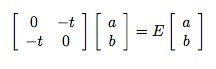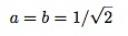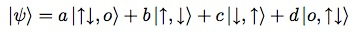## The Hubbard Model

I previously discussed high-temperature superconductivity in cuprates, and mentioned that the detailed mechanism is still controversial. However, what is widely agreed is that, as originally proposed by P.W. Anderson, a good model for these materials is the Hubbard Model (see this paper by Anderson for an entertaining and readable argument in favor of this point). And even if one doesn’t agree with that statement, the Hubbard Model is of enormous intrinsic interest, as perhaps the simplest model of interacting electrons on a lattice.

Despite its simplicity, physicists have not been able to solve for the behavior of the two or three-dimensional Hubbard model in the “thermodynamic limit” (for lattices with a very large number of sites and electrons). Coming up with a reliable approach to solving the Hubbard model has become a kind of holy grail of condensed matter theory.

Note that this is very different from the situation for the classical ferromagnetic Ising model, for which Onsager solved the two-dimensional version exactly in 1944 but where we do not have an exact solution in three dimensions. For the three-dimensional Ising model, we may not have an exact solution, but we understand extremely well the qualitative behavior, and can compute quantitative results to practically arbitrary accuracy. For the Hubbard model, we do not even have know what the qualitative behavior is for two or three dimensional lattices.

I will first describe the model in words, and then show you how to solve for the quantum statistical mechanics of the simplest non-trivial version of the model: the Hubbard model on a lattice with just two sites. I strongly believe that whenever you want to learn about a new algorithm or theory, you should start by solving, and understanding in detail, the smallest non-trivial version that you can construct.

The Hubbard Model Described With Words

The Hubbard model consists of a lattice of “sites,” plus a set of electrons that hop around from site to neighboring site in the lattice. Each electron has a spin that can point up or down.

A site can accommodate zero, one, or two electrons, but if two electrons are on the same site, they must have opposite spin (because electrons are fermions, and obey the Pauli exclusion principle). A configuration with two electrons of opposite spin on the same site has an increased energy, because electrons repel each other electrically. That energy cost is denoted U.

An electron can hop from one site to a neighboring site; when it does so, its spin doesn’t change. The “amplitude” (in quantum mechanics, you square amplitudes to get probabilities) for hopping is denoted -t.

It is sometimes useful to add two other refinements to the model. First we can add a magnetic field h. If h is positive, it will favor (i.e., reduce the energy of) configurations that have more electrons with spin up. Second, we can add a chemical potential μ . If μ is positive, it will increase the energy of configurations that have more electrons.

Quantum Statistical Mechanics

To solve for the statistical mechanics of an ordinary classical system, one weights every possible configuration of the system by a probability given by Boltzmann’s law:

P[configuration] = exp(-E[configuration]/T) / Z,

where T is the temperature and Z is just a normalization constant (called the “partition function.”)

In quantum statistical mechanics, one does exactly the same thing, except that one needs to solve for all the stationary solutions of the Schroedinger equation, including the energies of those states, and then weight those stationary states using Boltzmann’s law.

Solving the Two-Site Hubbard Model

I will now show you how to solve for the quantum statistical mechanics of the simplest non-trivial Hubbard model, one with two connected sites. By the way, the quantum mechanics of the two-site Hubbard model is useful for understanding the binding of molecules like H2 (take a look at Feynman’s Lectures on Physics, vol. III, chapter 10).

There are 16 possible configurations of electrons on two sites: one with no electrons, four with one electron (an up or down electron on each of the two sites), six with two electrons (one with two up electrons on different sites, one with two down electrons, and four with an up electron and a down electron), four with three electrons, and one with four electrons.

Because the electrons are conserved in number and cannot change their spin when they hop, these configurations split up into different unconnected groups, which we can solve separately. The configuration with no electrons is not connected to any other configuration, and has an energy of zero. The two configurations with an up electron are connected to each other, as are the two configurations with a single down electron. Let’s solve the stationary Schroedinger equation for the single up electron case.

We’ll write the wave-function in the form |ψ> = a |↑, o> + b |o ,↑>, where a and b are complex numbers that satisfy the normalization condition that the sum of their squares equals one, and |↑, o> is the configuration with the up electron on the first site with the second site open, while |o, ↑> is the other configuration with the up electron on the second site.

In general, the stationary Schroedinger Equation is H|ψ>=E|ψ>, where H is the Hamiltonian, and E is the energy. In our case, H is just a two-by-two matrix, and we need to solve the eigenvalue equationwhich has solutions ofwith E=-t, andwith E=t.

Here we already see the characteristic feature of quantum mechanics in the delocalized solutions. The spin is not definitely at one site or another, it is instead delocalized between them. There is a symmetric state with an energy of –t and an anti-symmetric state with energy t. If the system is in either one of these states, you will find that it is at one particular site with probability 1/2.

Now lets move on to the slightly more complicated computation for the configurations with two electrons. If they are both pointing up or both pointing down, there is nothing to do: we just have one configuration where they are on different sites with energy 0.

However, there are four configurations with one up spin and one down spin, and these configurations are connected to each other, so let’s write the wave-function aswhere I hope by now the notation is self-explanatory. Solving the Schroedinger equation for the configurations with one up and one down electron amounts to solving the eigenvalue equation.

I won’t work through the details of solving this equation in general; it’s simply a matter of straightforward mathematics to find the four solutions and their energies.

I will just make one observation, which is that the lowest energy state with one up and one down electron has a lower energy than the states obtained with two electrons of the same spin. Physically, this occurs because the electrons now have the ability to hop back and forth. This effect causes an anti-ferromagnetic tendency (spins on neighboring sites tend to want to point in opposite directions). So one thing that is agreed upon generally by theorists is that the Hubbard model with one electron per site should be an anti-ferromagnet at low enough temperatures.

The states obtained from configurations with three electrons are easy to work out; they have energies of U-t or U+t, and the state with four electrons has an energy of 2U.

Again, the overall statistical mechanical behavior is obtained by weighting these states with the appropriate Boltzmann factor. So there are two sources of randomness; the quantum randomness and then the statistical mechanical randomness layered on top of that. One important technical point: if you want to work at a particular density of electrons, you need to adjust the chemical potential appropriately.

Hopefully, I have given enough details so that you can at least understand how to set up the computations for the Hubbard Model for a larger lattice. Of course, if one tries to solve the problem as we did here for larger lattices, one quickly runs into enormous computational problems. There will be a number of connected configurations which grows exponentially with the number of sites, so that one will need to diagonalize an exponentially large matrix. Ouch.

So one needs more sophisticated methods. I’ll talk about some ideas that I and others have tried in the future. If you’re impatient, you can look at the following two papers now (or look at the references in Anderson’s paper already mentioned above):

Thermodyamics of the Infinite-U Hubbard Model

Onsager Reaction Terms for Quantum Many-Body Systems: Application to Antiferromagnetic and Superconducting Order in the Hubbard Model

### 4 Responses to “The Hubbard Model”

1.smm Says:

i was just turned on to your site by a reference from “information processing.” thanks for this nice tutorial. i’m looking forward to future installments.

2.Jonathan Yedidia Says:

Thanks for your comment. For those not in the know, “Information Processing” is Steve Hsu’s excellent blog; you should check it out! Steve and I were Junior Fellows together in the early 90’s at Harvard’s Society of Fellows.

3. Computing Free Energies « Nerd Wisdom Says:

[…] you’re interested in quantum systems like e.g. the Hubbard model, the expansion approach has the advantage that it can also be applied to them; see my paper with […]

4.Xinguo Ren Says:

this is really a nice tutorial of the two-site Hubbard model. The relevance of this model to the hydrogen molecule dissoication problem is of great interest. The problem is essentially how to correctly discribe the ground state as U/t increases. This poses a big problem to Kohn-Sham density functional theory, which simply can’t discribe this dissociation process. Many fundamental problems of KS-DFT can be already be understood from such a simple two-site model.